Next: 6.4 Reaction-Diffusion Model Up: 6. Negative Bias Temperature Previous: 6.2 The NBTI Time

Subsections

# 6.3 Physical Mechanisms of NBTI

Although the effect of bias temperature instability has been reported more than 40 years ago by several groups [83,89,90] there is still much controversy about the physical mechanisms behind the degradation and the exact causes for BTI are not yet fully understood. However, broad agreement has been found that when MOSFETs are stressed with a constant gate voltage at an elevated temperature, positive charge builds up either at the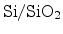interface or in the gate oxide layer. This charge leads to degradation of the transistor parameters.

One of the problems researchers face is that the exact transition from crystalline silicon to amorphous silicon-dioxide and the possible defects are not totally clear. Chapter 3 gives an overview of the resent research results concerning this interface and its defects.

In this section the proposed physical mechanisms that lead to BTI are presented and their validity discussed.

## 6.3.1 Basic Experimental Set-Up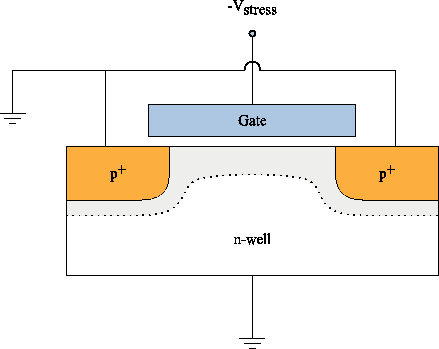The set-up for the observation of negative bias temperature (NBT) degradation of either a MOSFET or an MOS capacitor is schematically depicted in Figure 6.2. The substrate and in the case of the MOSFET also the source and drain contacts are grounded, while the gate is negatively biased. These bias conditions are applied at elevated temperatures, typically ranging between 100 and 200^C, for a certain period of time.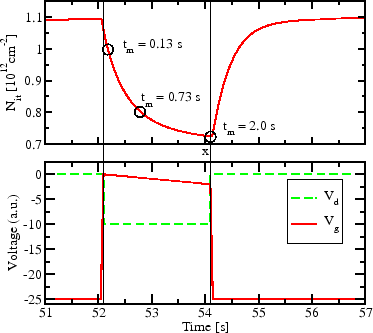To extract the level of degradation the stress is interrupted several times for short measurement cycles. During these measurement intervals typically the threshold voltage is measured performing a gate voltage sweep while measuring the current at the slightly forward biased drain contact. The interface trap density can be extracted using the charge pumping (Section 4.1), DCIV (Section 4.2), or CV (Section 4.3) methods.

This is the basic experimental set-up which was formerly used to obtain NBTI data found in literature. The problem with this method is the strong recovery of NBTI induced damage during the first milliseconds or even microseconds after the stress is released. Figure 6.3 qualitatively illustrates the measurement error obtained due to the fast recovery of NBTI degradation. When the stress gate voltage is removed, a part of the degradation relaxes. As a consequence the measured degradation of figures of merit, such as the threshold voltage or the interface trap density, is strongly dependent on the delay between the end of the stress conditions and the time where the value of the degradation is measured. This fast transient effect has been shown by Ershov et al. in 2003  and led to the development of advanced measurement methods as described below.

## 6.3.2 Faster Determination of the Threshold VoltageTo reduce the delay between stress and measurement, Kaczer et al.  proposed an improved measurement method to reduce the amount of relaxation. Instead of performing a full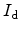vs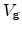sweep, the gate voltage is reduced from stress condition to a constant value around the initial threshold voltage,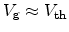. At this bias condition only one value for the drain current,, is measured. Using the unstressed/characteristic, an approximation for the new threshold voltage can be extracted by horizontally shifting the unstressed characteristic as outlined in Figure 6.4.

As only one drain current has to be obtained, instead of a range of drain currents for different gate biases, this approach is drastically faster and the error due to the fast recovery is reduced.

This technique still suffers from a measurement delay of some tens or hundreds of milliseconds. In order to completely remove the, normally unintentional, recovery during the measurement conditions on-the-fly measurement methods with zero delay have been proposed [93,94], which are discussed in the next sections.

## 6.3.3 Drain Pulsed Voltage

To completely remove the relaxation of the device degradation during measurement cycles, the gate voltage is constantly set to stress bias,. The drain voltage is normally kept at zero volt, but it is periodically pulsed with gate stress voltage,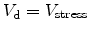. During the pulses the drain current is measured .

One concern using this method might be the following: although the stress gate voltage is never removed during measurement, biasing the drain contact with the same voltage still leads to a drastic change of the electric field at theinterface, especially close to the drain contact. As the electric field has been found to be one of the major driving forces of NBTI degradation, this change of the field will most likely lead to altered degradation behavior.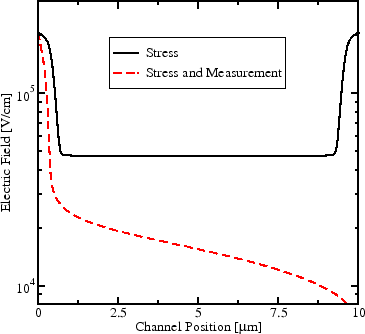Figure 6.5 depicts the electric field at theinterface of a MOSFET biased at symmetric stress conditions withand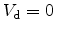V and also with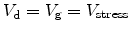. It can be clearly seen that the electric field component normal to the interface changes severely when applying a high drain voltage. This might invalidate the main argument for this measurement method, of not changing the degradation behavior during measurement.

The amount by which the electric field is altered during measurement depends strongly on the exact device geometry, the doping concentrations, and also the stress bias. Appropriate simulations have to be made to evaluate the impact on the electric field before using this measurement for device qualification.

Another problem of this method is the high channel current during measurement. It can lead to additional degradation mechanisms due to hot carrier stress (Section 5.1), as hot carrier injection into the oxide.

For circuit design the threshold voltage is in most cases of more interest than the drain current in saturation. The next on-the-fly method extracts the threshold voltage while keeping the gate stress approximately constant.

## 6.3.4 Gate Pulsed Voltage

By using the gate pulsed voltage (GPV) technique, the transconductance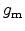, the drain current in the linear regime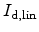, and the threshold voltage shift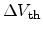can be extracted while keeping the gate stress nearly constant [93,94].

Using this method, the source and bulk contacts are grounded while the drain contact is slightly biased with typically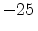mV. The stress at the gate contact is permanently applied and superimposed with pulses which are small compared to the stress voltage. With the small drain voltage, as compared to the drain pulsed voltage method, neither the problem of the severely changing interface electric field nor hot carrier degradation are imminent.

The transconductanceof the transistor, which is defined as the change in drain current as a result of a change of gate voltage, can then be extracted as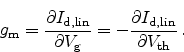(6.2)

From this relation also the threshold voltage can then be extracted.

## 6.3.5 Observation of Terminal Currents

Figure 6.6: Observed terminal voltages during stress. Abrupt changes in the terminal currents indicate an additional degradation mechanism such as dielectric breakdown (Section 5.2). Data are from IMEC.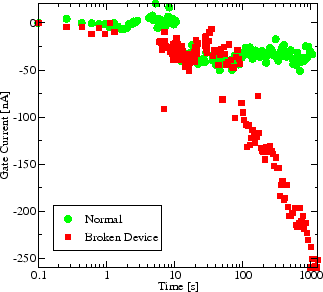Gate dielectric damage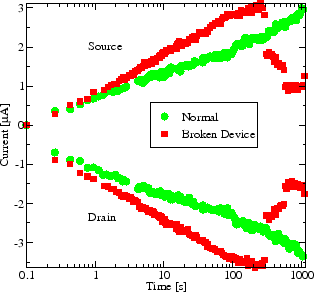Device damage

During stress tests it is often useful to measure the source, drain, and gate currents,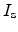,, and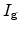. Devices which suffer from oxide breakdown during stress can then be detected asshould stay very low andshould be approximately equal to. Sudden changes in terminal voltages, as seen in Figure 6.6, are not typical for NBTI and suggest an additional degradation mechanism.

## 6.3.6 Importance of Initial Degradation

The effect of fast recovery of NBTI degradation has received much attention in recent years. Many groups proposed enhanced measurement schemes, as the on-the-fly measurements described above, to measure the true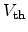shift free from any recovery effects [93,94,95,96,97,98]. Still, there is much controversy about different reported NBTI characteristics ranging from power-law exponents (Section 6.2) of around 0.16  or much smaller  to logarithmic dependence .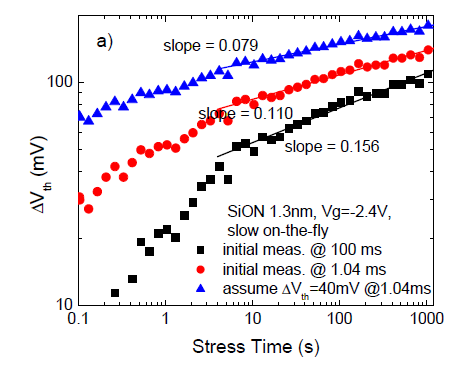As the major problem leading to the spread of the literature data Shen et al. identified the slow measurement of the initial degradation before stressing the device . The first measurement is compared to the subsequent measurements to extract the threshold voltage shift. As the first measurement already captures a certain amount of degradation this shift is underestimated. This underestimation is especially serious at short stress times and results in a kink as observed in a log-log plot and, probably more important, in completely different, apparent slopes in the power-law of the degradation.

Figure 6.7 depicts data from  showing the apparent threshold voltage shift over time in logarithmic scale. It can be seen that for long delay times of 100ms at the initial measurement, the kink in the power law is very pronounced. But as the initial measurement time is drastically reduced to 1.04ms, and therefore the accuracy of this measurement increased, the kink becomes less obvious. The authors showed that by assuming a shift of the threshold voltage of 40mV even at the fast initial measurement, and adding it to the following data they can achieve a clear power-law with the time exponent of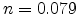. This slope varies drastically when the amount of initial degradation is not added and might explain the wide spread of the data reported in literature.

To conclude, not only the speed of the degradation measurements during stress is important in order to spot the fast transients during relaxation, but also the accuracy of the initial degradation measurement is of highest importance and can be improved by very high speed measurement methods .

## 6.3.7 pMOSFET vs. nMOSFET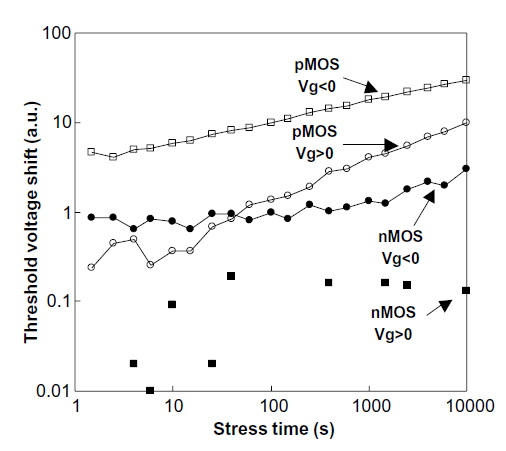The effect of bias temperature instability can be observed in both, p-channel and n-channel MOSFETs. However, Figure 6.8, presenting data from Huard et al. , shows that p-channel MOSFETs with negative gate voltage stress are much more susceptible to this kind of degradation.

It has been reported that for NBTI degradation channel cold holes are important [101,86]. As the n-channel MOSFET biased into accumulation also has holes at the surface of the substrate, the threshold voltage shift should be similar to p-channel MOSFETs. Therefore, the lack of holes can not be the cause for the different degradation behavior.

### 6.3.7.1 Charge States

One explanation for the different susceptibility of bias temperature stress (BTS) is based on the charge states of the interface traps,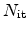, and the oxide traps,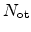.

Oxide traps are positively charged by hole trapping in both, the n- and p-channel MOSFET. Therefore their contribution to ashift should be comparable. The charge state of the interface traps, on the other hand side, depends on the Fermi-level as pointed out in Chapter 3. The threshold voltage of a MOSFET is reached when the surface of the substrate is driven into weak inversion. Therefore the charge state at the silicon-dielectric interface under inversion conditions is very important.

The Fermi-level at the interface of a p-channel MOSFET biased into inversion is below the mid-gap energy. As Figure 6.9(a) illustrates, in this regime the acceptor like trap levels are empty, and the donor like trap levels are partially filled, assuming the trap energy levels to be symmetrically aligned around mid-gap. The net charge resulting from this configuration is positive and leads to a total interface charge for p-channel MOSFETs of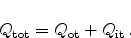(6.3)

Figure 6.9: Energy diagram of a (a) p-channel MOSFET and an (b) n-channel MOSFET at theinterface in weak inversion. The Fermi-level of the left substrate is energetically located in the donor trap resulting in a positive net charge at the interface. The Fermi-level of the right substrate, in contrast, is energetically located in the acceptor trap leading to negative interface charge.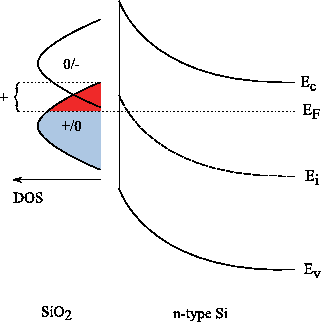p-channel MOSFET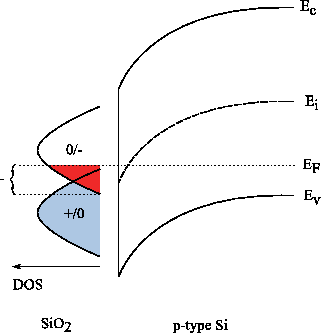n-channel MOSFET
For the n-channel MOSFET the situation is completely different. Figure 6.9(b) illustrates the energy diagram of an nMOSFET in inversion. Here, the Fermi-level at the interface is located above the mid-gap energy. The donor like trap levels in the lower half of the band gap are therefore completely filled and the acceptor like trap levels are partially filled. The result is a negative charge due to the interface traps which leads to a total interface charge for n-channel MOSFETs of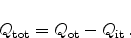(6.4)

In p-channel MOSFETs, both, the interface and the oxide traps contribute to the threshold voltage shift. In n-channel devices the charge state of the interface traps reduces the shift introduced by the oxide traps and the effect of NBTI is therefore less pronounced in n-channel MOSFETs.

### 6.3.7.2 Availability of Hydrogen

Another possible explanation for the different degradation behavior can be found in the model of Tsetseris et al. . As described in Section 6.5, the model proposes the importance of atomic hydrogen,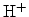, for weakening and breaking of Si-H bonds at the interface. These protons originate from the substrate of the transistor and are initially bond to dopants, usually phosphorus in the case of a pMOS (P-H bonds).

The activation energy for dissociation of P-H bonds is usually very high. Only in the depletion region the bonds are weakened and can be cracked more easily. During NBT stress a p-channel MOSFET is driven into inversion and a depletion region is formed in the substrate. In this region the hydrogen atoms can be dissociated and cause degradation at the interface. For positive stress no depletion region is formed. Therefore no hydrogen can be provided by dissociating P-H bonds, and the level of degradation is reduced.

In the n-channel MOSFET the substrate is typically doped with boron and hydrogen can only be supplied from B-H bonds. As their binding energy is much higher, also in depletion regions, n-channel MOSFETs are much less susceptible to bias instability, no matter which polarity the stress voltage has.

### 6.3.7.3 Work Function Difference

Another reason for different degradation behavior of p- and n-channel MOSFETs might be found in the fact that for a given oxide electric field the gate voltages differs .

The Fermi-level of a p-type poly gate lies approximately at the valence band edge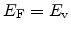while the Fermi-level of an n-type poly gate is located at the conduction band edge energy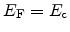. For NBT stress the p-type poly gate of the p-channel device is depleted while the n-type poly gate of the n-channel device is driven into accumulation.

Oxide and interface charges (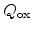and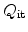) generated by NBTI degradation further shift the relevant gate voltages as described in Section 2.2.1.

Due to this asymmetry for n- and p-type gate contacts, the oxide electric fields are not equal for a given gate voltage and at a given oxide electric field the gate voltages differ. This has to be considered when comparing the two types of transistors.

## 6.3.8 Influence of Channel Carrier Transport

During NBT stress conditions the voltages of substrate, source, and drain contacts are typically equal (Section 6.3.1) or close to equal (Section 6.3.4). Under these conditions, the charge transport in the channel is negligible and so is its impact during NBTI investigations. The only displacement of channel carriers, holes in case of NBTI on p-channel MOSFETs, is due to thermal activity.Next: 6.4 Reaction-Diffusion Model Up: 6. Negative Bias Temperature Previous: 6.2 The NBTI Time

R. Entner: Modeling and Simulation of Negative Bias Temperature Instability